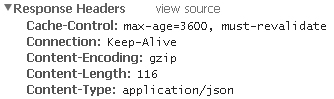# 将PHP程序中返回的JSON格式数据用gzip压缩输出的方法

更新时间：2016年03月03日 15:52:23   作者：傲雪星枫

1.使用压缩与不使用压缩的HTTP输出比较2.开启gzip

```sudo a2enmod deflate
sudo /etc/init.d/apache2 restart

```

```sudo a2dismod deflate
sudo /etc/init.d/apache2 restart

```

3.设置需要gzip压缩输出的类型

json的输出类型是application/json，所以可以这样设置

```<IfModule mod_deflate.c>
</IfModule>

```
```<?php
\$data = array(
array('name'=>'one','value'=>1),
array('name'=>'two','value'=>2),
array('name'=>'three','value'=>3),
array('name'=>'four','value'=>4),
array('name'=>'five','value'=>5),
array('name'=>'six','value'=>6),
array('name'=>'seven','value'=>7),
array('name'=>'eight','value'=>8),
array('name'=>'nine','value'=>9),
array('name'=>'ten','value'=>10),
);

echo json_encode(\$data);
?>

```4.单个json使用gzip压缩输出

```<?php
ob_start('ob_gzhandler');

\$data = array(
array('name'=>'one','value'=>1),
array('name'=>'two','value'=>2),
array('name'=>'three','value'=>3),
array('name'=>'four','value'=>4),
array('name'=>'five','value'=>5),
array('name'=>'six','value'=>6),
array('name'=>'seven','value'=>7),
array('name'=>'eight','value'=>8),
array('name'=>'nine','value'=>9),
array('name'=>'ten','value'=>10),
);